Author: Oscar Cronquist Article last updated on September 12, 2019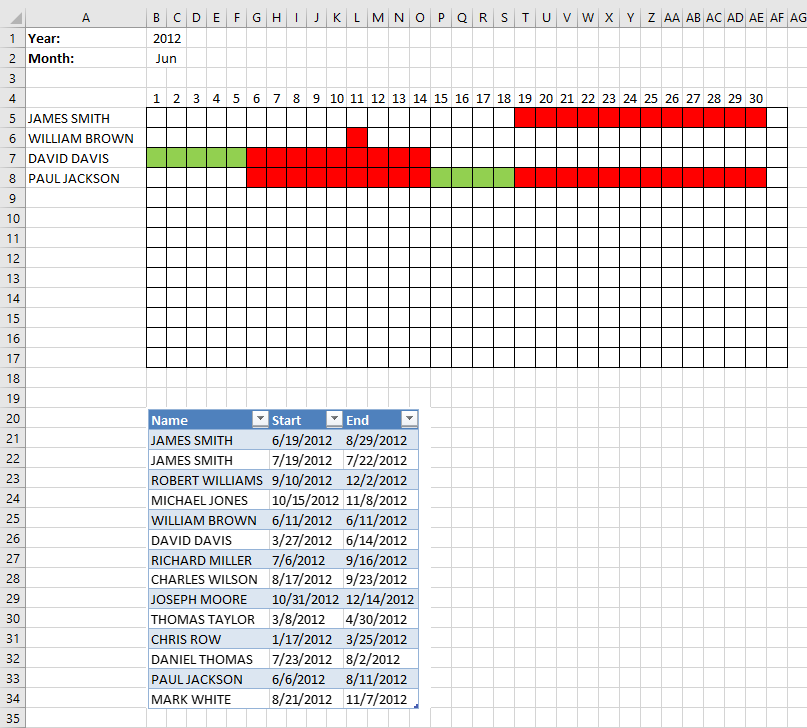I will in this article demonstrate a calendar that automatically highlights dates based on date ranges, the calendar populates names and corresponding dates based on the month and year selected by the user.

This is a new version of Visualize date ranges in a calendar. The workbook in this article lets you enter names and date ranges in an Excel defined Table. It allows you to add or delete names and date ranges without changing the cell references in the formulas.

Duplicate names are allowed, select year and month, days in that month are automatically calculated (row 4) and displayed accordingly.

Names whose date ranges are present in the selected month are displayed in cell range A5:A17. Dates are red if they overlap with another date range. This workbook contains no VBA code.

### What you will learn in this article

1. Create a dynamic monthly calendar.
2. Create a calendar that highlights date ranges green and overlapping date ranges red.
3. List names accordingly based on the corresponding date ranges.
4. Build Conditional formatting formulas that highlight cells based on name and date.
5. Build a formula that extracts names based on a year and month.

### How this worksheet works

The animated image above shows when I select a month and the calendar instantly displays the appropriate names and dates based on the date ranges below the calendar.

Enter a value in cell B1 to change year, use the drop down list in cell B2 to select the month.

### How I created this worksheet

#### Data list validation in cell B2

1. Select cell B2
2. Go to tab "Data"
3. Click "Data Validation" button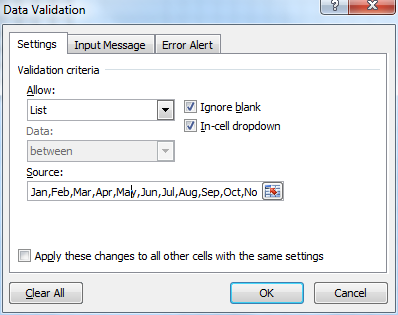4. Select List
5. Type: Jan,Feb,Mar,Apr,May,Jun,Jul,Aug,Sep,Oct,Nov,Dec in Source:
6. Click OK button

#### Calculate first date in selected month in cell D1

Formula:

=DATE(B1, MATCH(B2, {"Jan", "Feb", "Mar", "Apr", "May", "Jun", "Jul", "Aug", "Sep", "Oct", "Nov", "Dec"}, 0), 1)

#### Calculate last date in selected month in cell D2

Formula:

=DATE(B1, MATCH(B2, {"Jan", "Feb", "Mar", "Apr", "May", "Jun", "Jul", "Aug", "Sep", "Oct", "Nov", "Dec"}, 0)+1, 1)-1

#### Hide cell values in cell range D1:D2

These steps shows you how to hide values in a cell by applying cell formatting, the value is still there but you can't see it.

1. Select cell range D1:D2
2. Press Ctrl + 1
3. Go to "Number" tab
4. Click "Custom"
5. Type ;;;
6. Press OK

#### Calculate dates

Formula in cell B4:

=D1

Formula in cell C4:

=IF(MONTH(\$B\$4+COLUMN(A1))<>MONTH(\$D\$2), "", B4+1)

Copy cell C4 and paste to cell range D4:AF4.

#### Create an Excel defined Table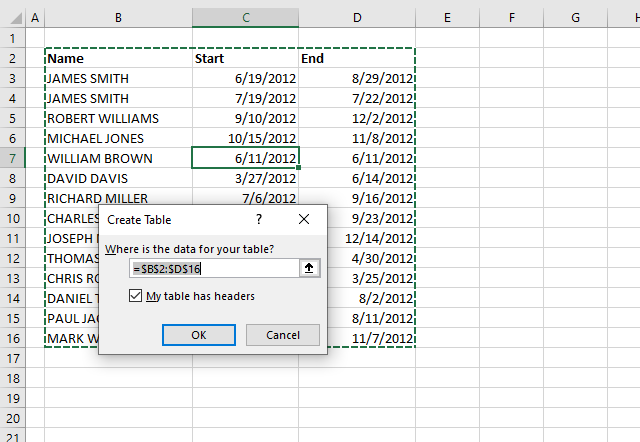1. Select any cell in the data set that contains names and date ranges.
2. Press CTRL + T to open the "Create Table" dialog box.
3. Click OK button.

#### Filter names in column A

Array formula in cell A5:

=IFERROR(INDEX(Table1[Name], SMALL(IF((Table1[Start]<=\$D\$2)*(Table1[End]>=\$D\$1), MATCH(ROW(Table1[Start]), ROW(Table1[Start]), 0), ""), ROW(A1))), "")
1. Copy above array formula
2. Paste in formula bar
3. Press and hold Ctrl + Shift
4. Press Enter
5. Copy cell A5 and paste to cell range A6:A17

#### Explaining array formula in cell A5

Step 1 - Identify records that overlap selected month

The less than and greater than signs are logical oerpators that allows you to compare the date ranges saved in the Excel defined Table to the hidden dates in cell D1 and D2.

Cell D1 contains the first date in the selected month and cell D2 contains the last date of the selected dates.

(Table1[Start]<=\$D\$2)*(Table1[End]>=\$D\$1)

becomes

({41079; 41109; 41162; 41197; 41071; 40995; 41096; 41138; 41213; 40976; 40925; 41113; 41066; 41142}<=41090)*({41150; 41112; 41245; 41221; 41071; 41074; 41168; 41175; 41257; 41029; 40993; 41123; 41132; 41220}>=41061)

becomes

{TRUE; FALSE; FALSE; FALSE; TRUE; TRUE; FALSE; FALSE; FALSE; TRUE; TRUE; FALSE; TRUE; FALSE}*{TRUE; TRUE; TRUE; TRUE; TRUE; TRUE; TRUE; TRUE; TRUE; FALSE; FALSE; TRUE; TRUE; TRUE}

and returns

{1;0;0;0;1;1;0;0;0;0;0;0;1;0}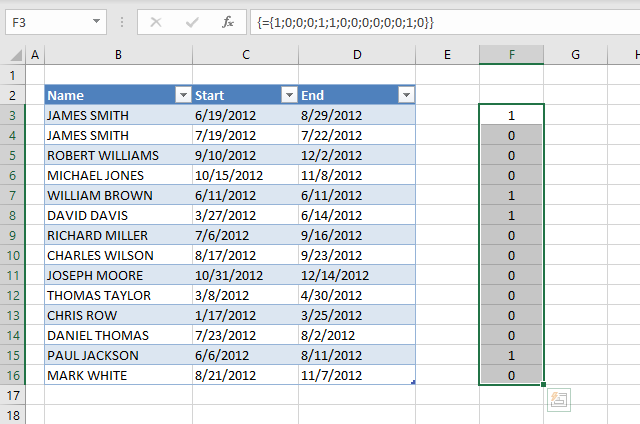The position of each value matches the records in the Excel defined Table, for example, 1 indicates that the first record overlaps the select month June and year 2012.

0 (zero) tells us that the record does not overlap the selected year and month.

Step 2 - Convert boolean values to corresponding relative row number

The IF function lets you use a logical expression to determine which argument to return: value_if_true or value_if_false.

IF(logical_test, [value_if_true], [value_if_false])

IF((Table1[Start]<=\$D\$2)*(Table1[End]>=\$D\$1), MATCH(Table1[Start], Table1[Start], 0), "")

becomes

IF({1;0;0;0;1;1;0;0;0;0;0;0;1;0}, MATCH(ROW(Table1[Start]), ROW(Table1[Start]), 0), "")

To create an array of numbers starting from 1 to the number of records in the Excel defined Table I use the MATCH and ROW functions.

The ROW function returns an array of numbers representing the row number for each record, however they don't start with 1 in most cases.

The MATCH function lets you convert the array to a sequence of numbers that start with 1.

IF({1;0;0;0;1;1;0;0;0;0;0;0;1;0}, MATCH(ROW(Table1[Start]), ROW(Table1[Start]), 0), "")

becomes

IF({1;0;0;0;1;1;0;0;0;0;0;0;1;0}, MATCH({3; 4; 5; 6; 7; 8; 9; 10; 11; 12; 13; 14; 15; 16}, {3; 4; 5; 6; 7; 8; 9; 10; 11; 12; 13; 14; 15; 16}, 0), "")

becomes

IF({1;0;0;0;1;1;0;0;0;0;0;0;1;0}, {1; 2; 3; 4; 5; 6; 7; 8; 9; 10; 11; 12; 13; 14}, "")

and returns

{1;"";"";"";5;6;"";"";"";"";"";"";13;""}.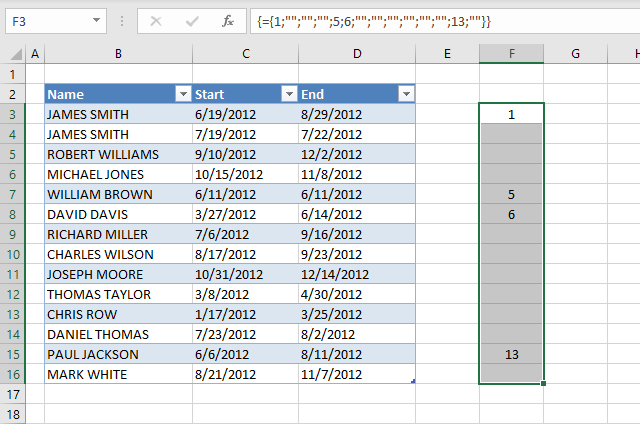The image above shows the array next to the Excel defined Table. The array contains the relative row number of each record that overlaps the selected year and month.

Step 3 - Extract k-th smallest row number

In order to extract a new value in each cell I use the SMALL function with a relative cell reference that changes automatically when I copy the cell to cells below.

SMALL(array, k)

SMALL(IF((Table1[Start]<=\$D\$2)*(Table1[End]>=\$D\$1), MATCH(Table1[Start], Table1[Start], 0), ""), ROW(A1))

becomes

SMALL({1;"";"";"";5;6;"";"";"";"";"";"";13;""}, ROW(A1))

becomes

SMALL({1;"";"";"";5;6;"";"";"";"";"";"";13;""}, 1)

and returns 1.

Step 4 - Return name

The INDEX function returns a value from a given cell range based on a row and column number.

INDEX(Table1[Name], SMALL(IF((Table1[Start]<=\$D\$2)*(Table1[End]>=\$D\$1), MATCH(Table1[Start], Table1[Start], 0), ""), ROW(A1)))

becomes

INDEX(Table1[Name], 1)

and returns the first value in column Name which is James Smith.

Step 5 - Remove errors

When values run out the formula returns an error, the IFERROR function removes the errors and returns a blank cell instead.

For example, in cell A9 the formula becomes:

IFERROR(INDEX(Table1[Name], SMALL(IF((Table1[Start]<=\$D\$2)*(Table1[End]>=\$D\$1), MATCH(Table1[Start], Table1[Start], 0), ""), ROW(A5))), "")

becomes

IFERROR(#NUM!, "")

and returns a blank.

#### Red conditional formatting formula

Conditional formatting formula applied to cell range B5:AF17:

=IF((INDEX(INDIRECT("Table1[Start]"), SMALL(IF(\$A5=INDIRECT("Table1[Name]"), MATCH(ROW(INDIRECT("Table1[Start]")), ROW(INDIRECT("Table1[Start]"))), ""), COUNTIF(\$A\$5:\$A5, \$A5)))<=B\$4)*((INDEX(INDIRECT("Table1[End]"), SMALL(IF(\$A5=INDIRECT("Table1[Name]"), MATCH(ROW(INDIRECT("Table1[Start]")), ROW(INDIRECT("Table1[Start]"))), ""), COUNTIF(\$A\$5:\$A5, \$A5)))>=B\$4)), SUMPRODUCT((INDIRECT("Table1[Start]")<=B\$4)*(INDIRECT("Table1[End]")>=B\$4))>1, FALSE)

#### Green conditional formatting

Conditional formatting formula applied to cell range B5:AF17:

=(INDEX(INDIRECT("Table1[Start]"), SMALL(IF(\$A5=INDIRECT("Table1[Name]"), MATCH(ROW(INDIRECT("Table1[Start]")), ROW(INDIRECT("Table1[Start]"))), ""), COUNTIF(\$A\$5:\$A5, \$A5)))<=B\$4)*((INDEX(INDIRECT("Table1[End]"), SMALL(IF(\$A5=INDIRECT("Table1[Name]"), MATCH(ROW(INDIRECT("Table1[Start]")), ROW(INDIRECT("Table1[Start]"))), ""), COUNTIF(\$A\$5:\$A5, \$A5)))>=B\$4))